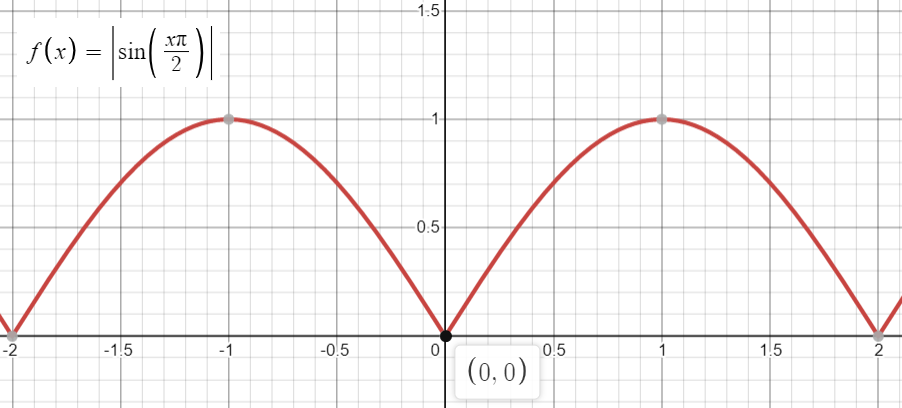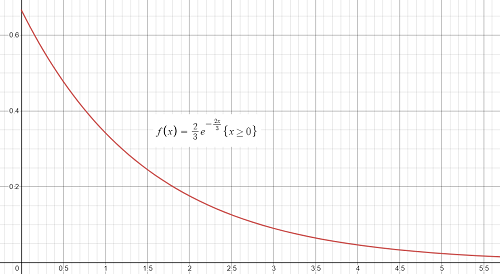# Non Negative Function

## What is a Non Negative Function?

A non negative function has function values equal to or greater than zero (i.e., f(x) ≥ 0). The domain (inputs) of the function can be negative, but the outputs (y-values) must be zero or greater in order for the function to be classified as non-negative. A positive function is similar, except that it does not include zero in its range.

An example of a non negative function is shown on the following graph of a sine function:Note the labeled point [0, 0]: the function values are zero an infinite number of times and all other values are always positive.

Classifying non negative functions might seem like a pedantic exercise, but these functions play an important role in calculus, real analysis, and many other branches of mathematics. If a function is non-negative, then its integral is the area between two points a and b. If that are is equal to 1, then the integral is the probability density function.A probability density function is a non negative function with an area under the curve equal to 1.

As another example, let’s say we had an integrable function on [0, ∞), its limit at infinity might not exist, but if the integrable function is non negative, and if any of the first four derivatives are bounded in a neighborhood of each relative maximum of f, then its limit does exist .

## References

Graphs: Desmos.
 Dix, J. (2013). Existence of the limit at infinity for a function that is integrable on the half line. Rose-Hulman Undergraduate Mathematics Journal. Spring.

CITE THIS AS:
Stephanie Glen. "Non Negative Function" From StatisticsHowTo.com: Elementary Statistics for the rest of us! https://www.statisticshowto.com/non-negative-function/